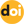The peer-reviewed scientific and technology journal. ISSN: 0204-3327

# Analytical estimations of pulse parameters in the modified integral neutron kinetics model for pulsed reactor and subcritical blockhttps://doi.org/10.26583/npe.2016.2.09

### UDC: 621.039.5

Application of modified integral neutron kinetic model to calculate principal characteristics of pulsed coupled reactor system consisting of pulsed reactor and subcritical block is discussed. The model is based on the use of respective time-dependent kernels of integral equation for reactor power and space-time Green’s function for the subcritical block. It is possible to reduce the set of integral equations to the set of elementary algebraic and first-order differential equations by using exponential approximation of the kernels and the Green’s function.

Approximations of «inertialess» reactivity dumping and jump reactivity boost on prompt neutrons are used as the «reactivity - power» feedback in order to close the mathematical model. This allows integrating corresponding kinetic equations in analytical form notwithstanding the fact that the kinetic equation for reactor is nonlinear.

Analytical relations allowing estimating basic characteristics of the system such as energy and maximum pulse power in the reactor and in the subcritical block with accuracy sufficient in engineering practices were obtained.

The performed calculations showed applicability of the analytical dependences of energy characteristics of the system on the impact coefficient of subcritical block on the reactor, on the lifetime of neutrons in the reactor and on the «time» constant of the block for fixed value of pulse energy in the reactor. The obtained ratio is valid for the reactor within the whole range of variation of system parameters while for the subcritical block it is correct only for the system operated with fast neutron spectrum in the reactor and with thermal neutron spectrum in the subcritical block when the so-called «delta» approximation of the reactor pulse is realized. In the case when such approximation is not valid the «Gaussian» approximation to the shape of the reactor pulse is applied for which more accurate analytical formulas were also obtained for estimation of maximum pulse energy in the block. These formulas depend on the ratio of duration of start-up period of the pulsed coupled reactor system to the value of «time» constant of the subcritical block and are correct for the systems with similar neutron spectra.

The obtained analytical relations can be applied for optimization of parameters of coupled reactor-laser systems.

1. Gulevich A.V., Kukharchuk O.F., Pashin E.A., Polevoy V.B. Modifitsirovannaya model neytronnoy kinetiki reaktornolazernogo ustroystva [A modified model of neutron kinetics of reactorlaser device]. Preprint FJEI-2264, Obninsk, 1992, 20 p. (in Russian).
2. Gulevich A.V., Dyachenko P.P., Zrodnikov A.V., Kukharchuk O.F. Svyazannye reaktornye sistemy impulsnogo deystviya [Pulsed coupled reactor systems]. Moscow. Energoatomizdat Publ., 2003, 360 p. (in Russian).
3. Bell D., Glasstone S. Teoriya yadernyh reaktorov [Nuclear reactor theory]. Moscow. Atomizdat Publ., 1974, 493 p. (in Russian).
4. Shabalin E.P. Impulsnye reaktory na bystrykh neytronah [Pulsed fast neutron reactors]. Moscow. Atomizdat Publ., 1976, 248 p. (in Russian).
5. Gulevich A.V., Kukharchuk O.F. Analiticheskie otsenki parametrov neytronnyh impulsov v lazernoj sisteme s nakachkoy ot impulsnogo reaktora [Analytical estimates of neutron pulses parameters in a laser system pumped by a pulsed reactor]. Izvestia Visshikh Uchebnikh Zavedeniy. Yadernaya Energetika. 1996, no. 1, p. 37 (in Russian).
6. Lomidze V.L. Impulsnye yadernye reaktory [Pulsed nuclear reactors]. Moscow. Znanie Publ., 1982, 63 p. (in Russian).
7. Rakitin V.I. Rukovodstvo po metodam vychislenij i prilozheniya MATHCAD [Calculation methods and MATHCAD application manual]. Moscow. Fizmatlit Publ., 2005, 246 p. (in Russian).
8. Kolesov V.F. Aperiodicheskye impusnye reactory [Aperiodic pulsed reactors]. V.1,2. Sarov. FGUP «RFNC-VNIIEF», 2007, 553 p. (in Russian).
9. Levakov B.G., Lukin A.V., Magda E.P. Impul’snye yadernye reaktory RFNC-VNIITF [Pulsed nuclear reactors of RFNC-VNIITF]. Snezhinsk. RFNC-VNIITF, 2002, 608 p. (in Russian).
10. Avery R. Theory of coupled reactors. 1958, Proc. of 2nd Int. Conf. on Peaceful Uses of Atomic Energy. Report No.1858.
11. Coupled Reactor Kinetics. Proc. of National Topical Meeting American Nuclear Society. Ed. C.Chezem, W.Kohler, Texas, 1967.
12. Komata M. On the Derivation of Avery’s Coupled Reactor Kinetics Equations. Nucl. Sci. and Eng., 1968, v. 38, p. 193.
13. Stevenson M., Gage S. Application of a Coupled Fission Mode Approach to Modular Reactor Kinetics. J. of Nucl. Ener., 1970, v. 24, iss. 1, p. 1.
14. Thayer G., Miley G., Jones B. Experimental Studies of Large Amplitude Transients in Weakly Coupled Cores. Trans. of Amer. Nucl. Soc., 1972, v. 15, iss. 2, p. 925.
15. Thayer G., Miley G., Jones B. An Experimental Study of Two Coupled Reactors. Nucl. Techn., 1975, v. 25, no. 1, р. 56.
16. Difilippo F., Waldman R. The Kinetics of a Coupled TwoCore Nuclear reactor. Nucl. Sci. Engng., 1976, v. 61, no. 1, p.60.
17. Kouvshinov M.I., Cherednik P.F., Ignatov I.I. Experimental Investigation of Coupled Systems Containing Pulsed Reactor BIR and Subcritical Assembly]. Voprosy Atomnoi Nayki i Techniki. Ser. Physics of Nuclear Reactors. 1988, no. 2, p. 3 (in Russian).
18. Takezawa H., Obara T., Gulevich A., Kukharchuk O. Criticality Analysis of Pulse Core and Laser Module Coupled Small Reactor with Low Enriched Uranium. Progress in Nuclear Energy. 2008, v. 50, iss. 26, p. 304.
19. Takezawa H., Obara T. New approach to spacedependent kinetic analysis by the integral kinetic model. Nucl. Sci. Eng. 2012, v. 171, p. 1.
20. Tuya D.; Obara T. Supercritical kinetic analysis in simplified system of fuel debris using integral kinetic model. Annals of Nuclear Energy. 2016, v. 91, p. 59.